Get instant live expert help with Excel or Google Sheets“My Excelchat expert helped me in less than 20 minutes, saving me what would have been 5 hours of work!”

#### Post your problem and you’ll get Expert help in seconds.

Your message must be at least 40 characters
Our professional Expert are available now. Your privacy is guaranteed.

# How to Generate Random Date Between Two Dates

We may need to randomly assign different groups to a set of people or select a sample of data for analysis out of an entire population data with the help of random numbers. Here we are going to learn how to generate a random date between two dates using RANDBETWEEN function.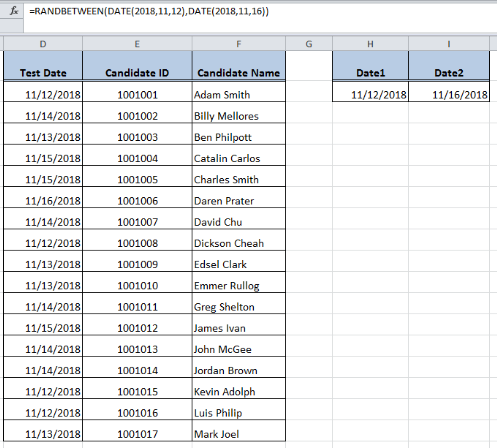Figure 1. Final result

## RANDBETWEEN Function

The RANDBETWEEN function returns a random numeric value between two specified numeric values. As dates are stored as serial numbers in Excel, therefore we can generate a random date between two dates using RANDBETWEEN function.

The generic syntax for RANDBETWEEN function is:

`=RANDBETWEEN(bottom, top)`

OR

`=RANDBETWEEN(date1, date2)`

In this example, we want to assign a random test date for a physical fitness test to a group of candidates. We have two date values (bottom and top date values). We want to assign a random date between those two dates to each candidate for this test. In Column D random test dates are required to generate, Column E contains candidates IDs and column F contains candidates names. Cell H2 contains the bottom value or Date1 and cell I2 contains the top value or Date2.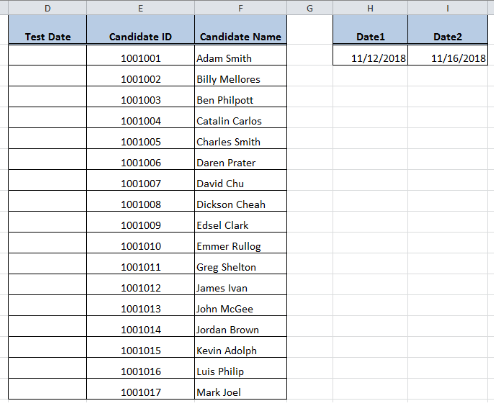Figure 2. Candidates_dataset

Excel RANDBETWEEN function accepts bottom and top value arguments either as cell reference or using DATE function.

## Entering Dates using Cell References

As per above example, we have the bottom and top value arguments as Date1 and Date2 in cell H2 and I2 respectively. We need to use these cell references to generate a random date between these given two dates using the following formula:

`=RANDBETWEEN(\$H\$2,\$I\$2)`

To get the accurate results of a random date between two dates, follow these points:

• Select targeted cells’ range in Column D > mouse right click > select “Format Cells” and choose desired date format to apply the format as date and press OK.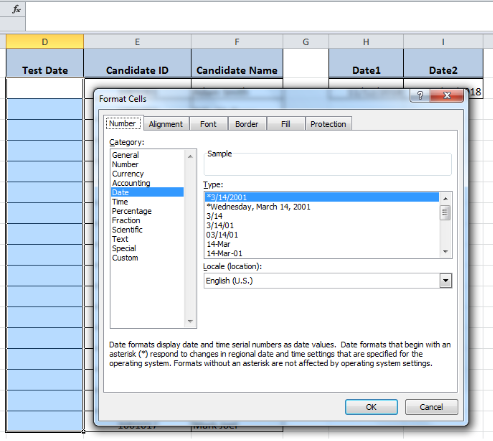Figure 3. Format Cells as Date

• In cell D2, apply RANDBETWEEN formula using cell references, such as `=RANDBETWEEN(\$H\$2,\$I\$2)`, and press Enter. Insert cell references as absolute references so that cell references do not change when copying this formula to other rows.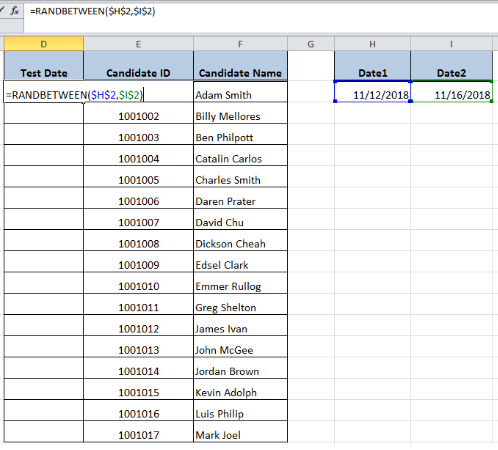Figure 4. Applying formula in cell D2

• Copy this formula to other rows or cells to generate a random date between two dates.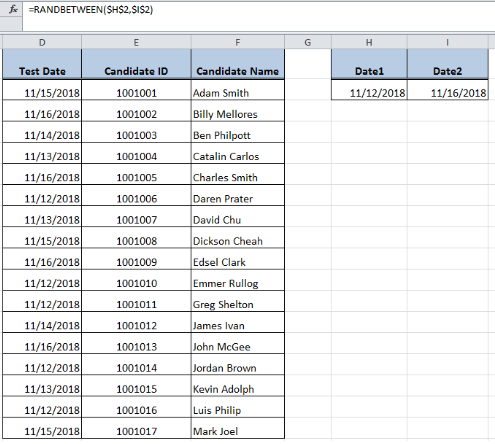Figure 5. Copying formula to other cells

• Once random dates are generated, this formula will generate new random dates whenever we edit any cell having this formula or open the worksheet again. To avoid this, simply copy the formulas and replace with values using Paste Special > Values feature.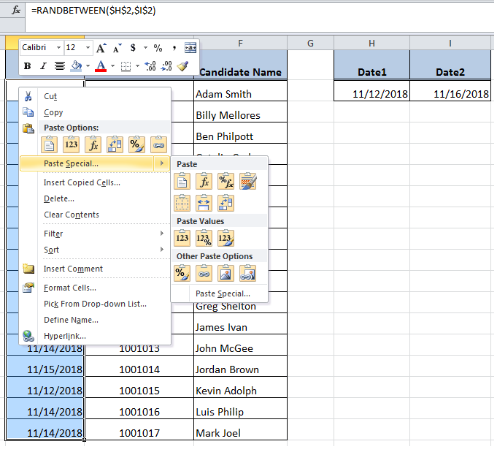Figure 6. Replace Formulas with Values

## Using Date function

Instead of using cell references, we can use Date function to enter dates as the bottom and top value arguments in RANDBETWEEN function. As our bottom and top date value arguments are 11/12/2018 and 11/16/2018 respectively, therefore we need to enter these dates using Excel Date function in the following formula:

`=RANDBETWEEN(DATE(2018,11,12),DATE(2018,11,16))`

Follow below steps to generate a random date between two dates:

• Format the targeted cells in column D as the date as explained above.
• Apply RANDBETWEEN formula in cell D2 using Date function, such as, `=RANDBETWEEN(DATE(2018,11,12),DATE(2018,11,16))` and press ENTER.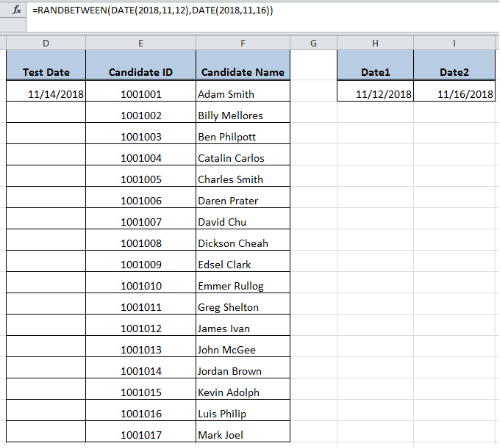Figure 7. Applying Formula with Date Function

• Then copy down the formula to other cells to generate a random date between two dates.Figure 8. Copying formula to other cells

• This formula will generate new random dates whenever any cell having the formula edits or worksheet opens again. To avoid this, simply copy the formulas and replace with values using Paste Special > Values feature as explained above.

## Instant Connection to an Expert through our Excelchat Service:

Most of the time, the problem you will need to solve will be more complex than a simple application of a formula or function. If you want to save hours of research and frustration, try our live Excelchat service! Our Excel Experts are available 24/7 to answer any Excel question you may have. We guarantee a connection within 30 seconds and a customized solution within 20 minutes.

### Did this post not answer your question? Get a solution from connecting with the expert.Another blog reader asked this question today on Excelchat:
Related blogs
Solution examplesI have multiple columns on my spread sheet and want to switch from A-Z to Z-A based on values in column A
Solved by V. H. in 38 minsConcat formula is incorrectly formatting the date in column C. Currently column B has dd/mm/yyyy and I require mm/dd/yyyy
Solved by Z. Y. in 31 minsexcel if x>=Y then display Y if x<= Y then display X or 0
Solved by K. U. in 18 minsi am trying to make a box change colors based on another boxes value. the issue is I need it to change based on multiple boxes depending on which one is most recent. example, box c4 is white unless box f4 is red, then it turns red as well, however if box g4 is green then c4 is no longer red but instead turns back to white.
Solved by C. Q. in 51 minsI have Mac Excel 2016. I need to sort a column in a way which is neither ascending nor descending, and it doesn't concern days of week or months. It won't let me create my own order in custom sort.
Solved by C. A. in 60 mins0 Followers

Most reacted comment
2 Comment authorsRecent comment authorsGuest
Andrew McCollum

I need some help. I am trying to use the RANDBETWEEN formula and it never executes. I am trying to use vales of a date that I have broken apart to year, month, day and then applying the formula to another cell to calculate it.
=RANDBETWEEN( DATE(X2,V2,W2), DATE(AA2,Z2,AB2)) It doesn’t work at all.

## Subscribe to Excelchat.coAnother blog reader asked this question today on Excelchat: